## Bell PolynomialTwo different Generating Functions for the Bell polynomials forare given by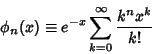orwhereis a Binomial Coefficient.

The Bell polynomials are defined such that, whereis a Bell Number. The first few Bell polynomials are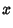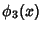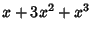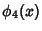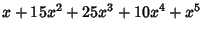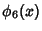Bell, E. T. Exponential Polynomials.'' Ann. Math. 35, 258-277, 1934.